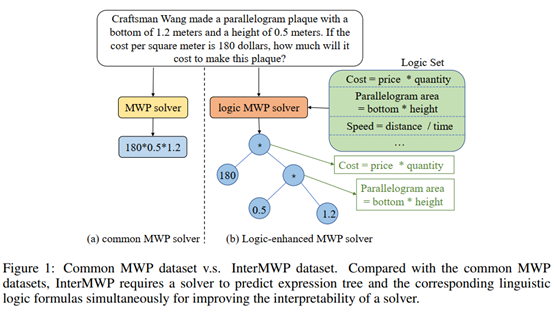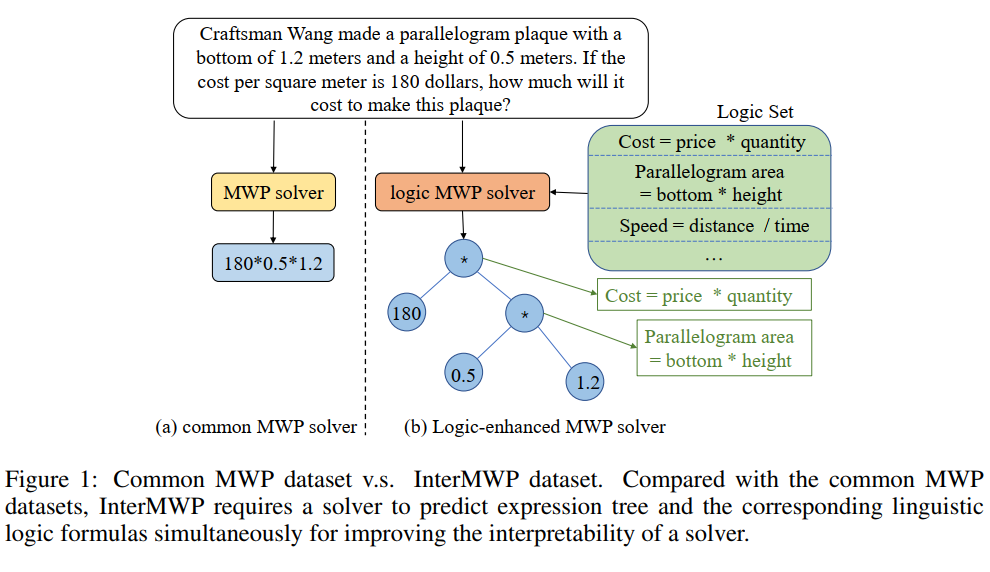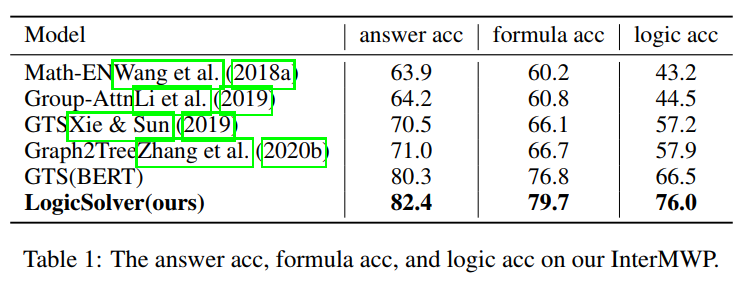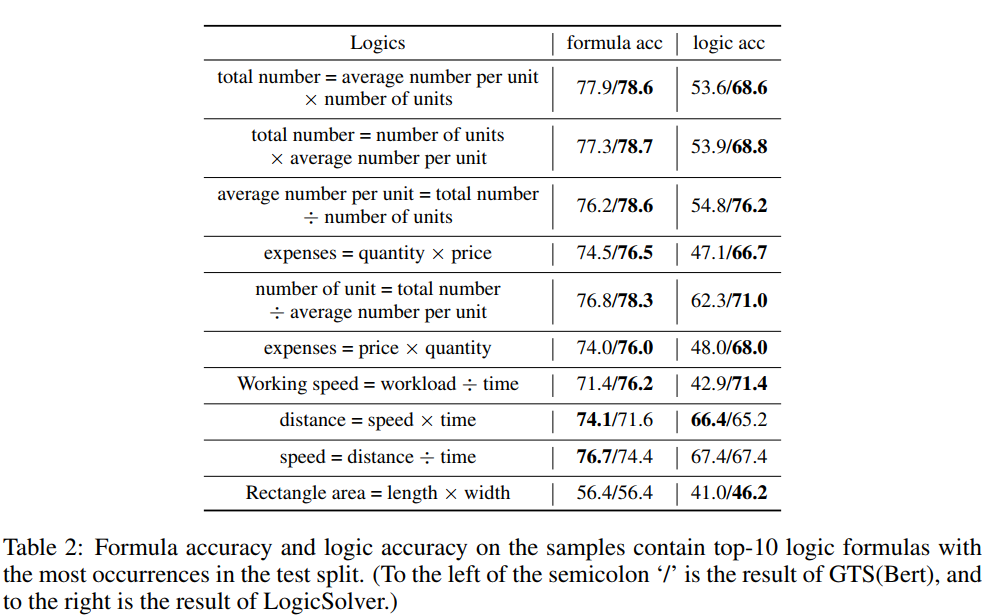Findings of EMNLP 2022
LogicSolver - Towards Interpretable Math Word Problem Solving with Logical Prompt-enhanced Learning
Zhicheng Yang, Jinghui Qin, Jiaqi Chen, Liang Lin and Xiaodan Liang
Findings of EMNLP 2022

Abstract

Recently, deep learning models have made great progress in MWP solving on answer accuracy. However, they are uninterpretable since they mainly rely on shallow heuristics to achieve high performance without understanding and reasoning the grounded math logic. To address this issue and make a step towards interpretable MWP solving, we first construct a high-quality MWP dataset named InterMWP which consists of 11,495 MWPs and annotates interpretable logical formulas based on algebraic knowledge as the grounded linguistic logic of each solution equation. Different from existing MWP datasets, our InterMWP benchmark asks for a solver to not only output the solution expressions but also predict the corresponding logical formulas. We further propose a novel approach with logical prompt and interpretation generation, called LogicSolver. For each MWP, our LogicSolver first retrieves some highly-correlated algebraic knowledge and then passes them to the backbone model as prompts to improve the semantic representations of MWPs. With these improved semantic representations, our LogicSolver generates corresponding solution expressions and interpretable knowledge formulas in accord with the generated solution expressions, simultaneously. Experimental results show that our LogicSolver has stronger logical formula-based interpretability than baselines while achieving higher answer accuracy with the help of logical prompts, simultaneously.

FrameworkExperimentConclusion

In this paper, to take a step towards interpretable MWP solving, we construct an interpretable math word problem dataset called InterMWP which consists of 11,495 MWP data and annotates interpretable logical formulas based on algebraic knowledge as the grounded linguistic logic of each solution equation. Different from existing MWP datasets, our InterMWP benchmark asks for a solver to not only output the solution expressions but also predict the corresponding logical formulas. We further propose LogicSolver which is enhanced by logical prompts and is able to generate corresponding solution expressions and interpretable knowledge formulas in accord with the generated solution expressions, simultaneously. Experimental results show that our LogicSolver has stronger logical formula-based interpretability than baselines while achieving higher answer accuracy with the help of logical prompts, simultaneously.